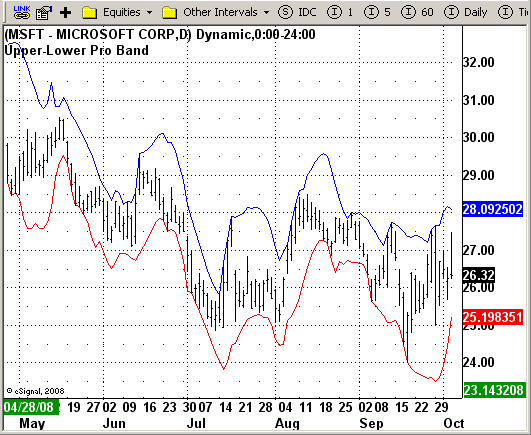# Upper Lower Pro Band

ICE Data Services -

Upper_LowerProBand.efs                                                                                              EFSLibrary - Discussion Board

File Name: Upper_LowerProBand.efs

Description:
Upper-Lower Pro Band

Formula Parameters:
Length: 14

Notes:
Bands define the upper and lower boundaries of a security's normal trading
range. A sell signal is generated when the security reaches the upper band
whereas a buy signal is generated at the lower band. The optimum percentage
shift depends on the volatility of the security--the more volatile, the larger
the percentage.

Upper_LowerProBand.efsEFS Code:

```/*********************************
Provided By:
eSignal (Copyright c eSignal), a division of Interactive Data
Formula Script (EFS) is for educational purposes only and may be
modified and saved under a new file name.  eSignal is not responsible
for the functionality once modified.  eSignal reserves the right
to modify and overwrite this EFS file with each new release.

Description:
Upper-Lower Pro Band

Version:            1.0  10/04/2008

Notes:
Bands define the upper and lower boundaries of a security's normal trading
range. A sell signal is generated when the security reaches the upper band
whereas a buy signal is generated at the lower band. The optimum percentage
shift depends on the volatility of the security--the more volatile, the larger
the percentage.

Formula Parameters:                     Default:
Length                                14

**********************************/

var fpArray = new Array();
var bInit = false;

function preMain(){
setPriceStudy(true);
setStudyTitle("Upper-Lower Pro Band");
setCursorLabelName("PU", 0);
setCursorLabelName("PL", 1);
setDefaultBarFgColor(Color.blue, 0);
setDefaultBarFgColor(Color.red, 1);

var x=0;
fpArray[x] = new FunctionParameter("Length", FunctionParameter.NUMBER);
with(fpArray[x++]){
setLowerLimit(1);
setDefault(14);
}
}

var xUpper = null;
var xLower = null;

function main(Length) {
var nState = getBarState();
var nUpper = 0;
var nLower = 0;

if (nState == BARSTATE_ALLBARS) {
if (Length == null) Length = 14;
}

if ( bInit == false ) {
var SumBars = Length * (Length - 1) * .5;
var SumSqrBars = (Length - 1) * Length * (2 * Length - 1) / 6;
xUpper = efsInternal("Calc_Upper", Length, SumBars, SumSqrBars);
xLower = getSeries(xUpper,1);
bInit = true;
}

nUpper = xUpper.getValue(0);
nLower = xLower.getValue(0);

if (nUpper == null || nLower == null) return;

return new Array (nUpper, nLower);
}

var xInit = false;
var xHigh = null;
var xLow = null;

function Calc_Upper(Length, SumBars, SumSqrBars){
var SH = 0.0;
var Sum1_2 = 0.0;
var SumY2 = 0.0;
var SL = 0.0;
var Sum1_1 = 0.0;
var SumY1 = 0.0;
var i = 0;

if(xInit==false){
xHigh = high();
xLow = low();
xInit = true;
}

if(xHigh.getValue(-Length) == null || xLow.getValue(-Length) == null) return;

for (i = 0; i < Length; i++){
Sum1_2 += i * xHigh.getValue(-i);
SumY2 += xHigh.getValue(-i);
Sum1_1 += i * xLow.getValue(-i);
SumY1 += xLow.getValue(-i);
}

var Sum2_2 = SumBars * SumY2;
var Num1_2 = Length * Sum1_2 - Sum2_2;
var Sum2_1 = SumBars * SumY1;
var Num1_1 = Length * Sum1_1 - Sum2_1;
var Num2 = SumBars * SumBars - Length * SumSqrBars;

SH = Num1_2 / Num2;
SL = Num1_1 / Num2;

var Value3 = 0.0;
var PU = 0.0;
var Value2 = 0.0;
var PL = 0.0;

for (i = 1; i <= Length; i++){
Value3=xHigh.getValue(-i + 1) + (SH * (i - 1));
Value2=xLow.getValue(-i + 1) + (SL * (i - 1));
if (i == 1){
PU=Value3;
PL=Value2;
}
if (Value3 > PU) PU = Value3;
if (Value2 < PL) PL = Value2;
}

return new Array (PU, PL);
}
```